### Home > CALC > Chapter 1 > Lesson 1.3.1 > Problem1-104

1-104.
1. Find the exact value(s) of x in the domain {x : 0 ≤ x ≤ 2π} if: Homework Help ✎

1.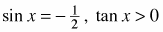.

2. cot x is undefined, cos x > 0.

3.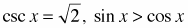.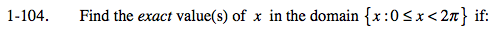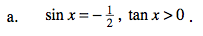$\text{sin}x=\frac{\text{opposite}}{\text{hypotenuse}},\text{ cos}x=\frac{\text{adjacent}}{\text{hypotenuse}},\text{ tan}x=\frac{\text{sin}x}{\text{cos}x}$

In which quadrant of the unit circle is sine negative and tangent positive?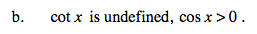Refer to the hint in part (a). And recall that cotangent is the reciprocal of tangent.

For cotx to be undefined, its denominator must equal 0.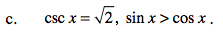Refer to the hint in part (a). And recall that cosecant is the reciprocal of sine.